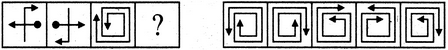Non Verbal Reasoning - Analogy - Discussion

Discussion :: Analogy - Section 1 (Q.No.10)

Each of the following questions consists of two sets of figures. Figures A, B, C and D constitute the Problem Set while figures 1, 2, 3, 4 and 5 constitute the Answer Set. There is a definite relationship between figures A and B. Establish a similar relationship between figures C and D by selecting a suitable figure from the Answer Set that would replace the question mark (?) in fig. (D).

10.

Select a suitable figure from the Answer Figures that would replace the question mark (?).

Problem Figures:                            Answer Figures:(A)     (B)      (C)     (D)                  (1)      (2)      (3)      (4)      (5)

 [A]. 1 [B]. 2 [C]. 3 [D]. 4 [E]. 5

Explanation:

The figure gets rotated through 180o.

 Animesh Sarkar said: (Jun 25, 2015) Why not answer A?

 Saksham Solanki said: (Aug 7, 2015) Explanation is wrong according to it the answer is 1 but correct answer is 2. Here is the correct explanation:- See in problem figures that B is a image formed by first generating the mirror image of A and then rotating it 180 degree (vertically) now same applies on C so, the answer is 2 which is totally satisfied the above mentioned conditions.

 Eshu said: (Sep 26, 2015) What is the process of rotating 180 degree vertically?

 Sreenu said: (Aug 21, 2016) It's actually rotated by 180 degrees first horizontally and then vertically.